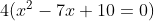# Algebra II : Polynomials

## Example Questions

1 2 11 12 13 14 15 16 17 19 Next →

### Example Question #10 : Write A Polynomial Function From Its Zeros

Which of the following equations belongs to a quadratic with zeros at x = 2 and x = 7?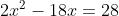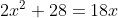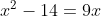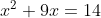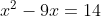Explanation:

The answer is.

A quadratic with zeros at x = 2 and x = 7 would factor to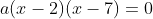, where it is important to recognize that a is the coefficient. So while you might be looking to simply expand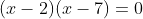to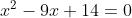, note that none of the options with a simple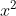term (and not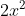) directly equal that simple quadratic when set to 0.

However, if you multiplyby 2, you get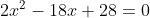. That’s a simple addition of 18x to both sides of the equation to get to the correct answer:.

### Example Question #11 : Write A Polynomial Function From Its Zeros

Which of the following equations represents a quadratic equation with zeros at x = 3 and x = -6, and that passes through point (2, -24)?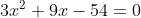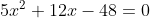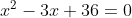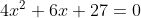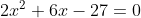Explanation:

When finding the equation of a quadratic from its zeros, the natural first step is to recreate the factored form of a simple quadratic with those zeros. Putting aside the coefficient, a quadratic with zeros at 3 and -6 would factor to: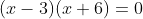So you know that a possible quadratic for these zeros would be: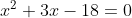Now you need to determine the coefficient of the quadratic, and that’s where the point (2, -24) comes in. That means that if you plug in x = 2, the result of the quadratic will be -24.  So you can set up the equation: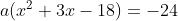, where a is the coefficient you’re solving for. And you know that this is true when x = 2, so if you plug in x = 2 you can solve for a: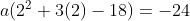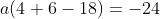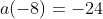So a = 3.  When you then distribute that coefficient of 3 across the original simple quadratic, you have: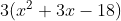So your quadratic is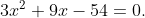### Example Question #11 : Write A Polynomial Function From Its Zeros

Which of the following equations belongs to a quadratic with zeros at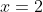and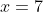?Explanation:

A quadratic with zeros at 2 and 7 would factor to, where it is important to recognize that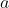is the coefficient. So while you might be looking to simply expand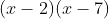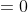to, note that none of the options with a simpleterm (and not) directly equal that simple quadratic when set to 0.

However, if you multiplyby 2, you get. That’s a simple addition of 18x to both sides of the equation to get to the correct answer:.

### Example Question #721 : Intermediate Single Variable Algebra

Which of the following represents a quadratic with zeros at 5 and 2 and that passes through point (1, 16)?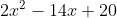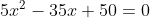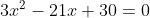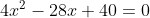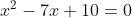Explanation:

When you're finding the equation of a quadratic given its zeros, a good start is to ignore the coefficient (you'll return to it later) and to construct the factored form of the quadratic using the zeros. Here with zeros of 5 and 2, that factored form would be: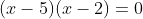That then means you can expand it to:From here, you can see that the answer choices all maintain the same general relationship as this simple quadratic, but have different coefficients. This is where you can use the point (1, 16) to your advantage. This means that if you plug in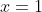to the quadratic, it will produce an answer of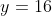. To solve this algebraically, return to using a coefficient in front of your entire quadratic. You'll then have: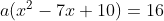Whereis the coefficient you can solve for knowing that the equation will produce a value ofwhen you input.  So plug that in and you can solve for the coefficient: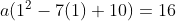Meaning that: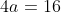So you know that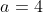.